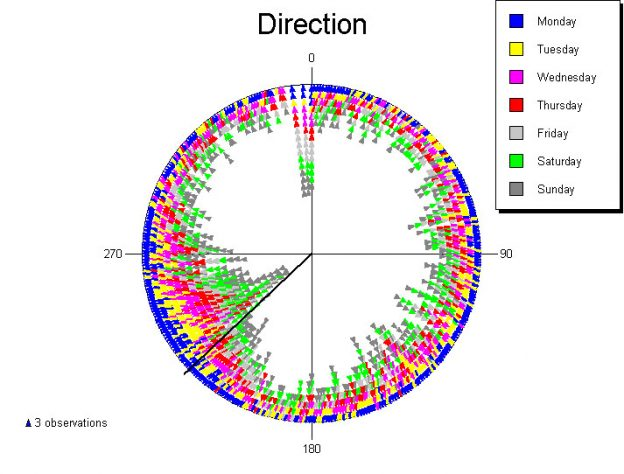# New Features in Oriana v.4

General Features

• New data type: lunar – Data are entered as dates but are treated as circular data based on the lunar month. This is useful when studying phenomena that might be related to lunar phase (e.g. turtles laying eggs during the full moon).
• Analyzing frequency data – Data can now be entered directly into the data editor as a frequency table, with one variable being the circular data and an associated Linear variable giving the frequency (number of observations for that circular measurement). These can then be used to produce histograms and basic stats.

Statistical Analyses

• Hotelling’s paired multisample test
• Moore’s paired multisample test (nonparametric)
• Hotelling’s test for weighted data
• Moore’s modified Rayleigh test for weighted data
• Second order statistical analysis: Grand mean angle, length and confidence limits
• Hotelling’s one sample second order test
• Moore’s modified Rayleigh test for second order analyses
• Hotelling’s two sample second order test
• Mardia’s two sample second order test (nonparametric)
• Hotelling’s paired sample second order test
• Moore’s paired sample second order test (nonparametric)

Data Handling

• Added option to save means and mean vector lengths to a file for further second order analysis.
• A new data transformation facility was added. Data entered or imported in radians can be converted to degrees for analysis. Also, linear data can be standardized and log or square root transformed.
• Added function to merge several Oriana data files into a single file

New Graph Options

• Added an option for circular plots to plot only the data between 0-180°, but spread around the full circumference of the circle. This type of graph is used in fields such as ophthalmology (doubled-angle plots).
• Added an option on circular-linear plots to plot the weighted mean as a centroid, along with an ellipse depicting the standard deviation of the data along the two major axes of the plot. This also is used in ophthalmological studies.
• Added option to have the degree symbol printed after the angle labels on graphs
• Added a new option for number of minor divisions on circular graphs (every 15°)
• Added plotting of the grand mean vector when subgroup means are included in histograms.